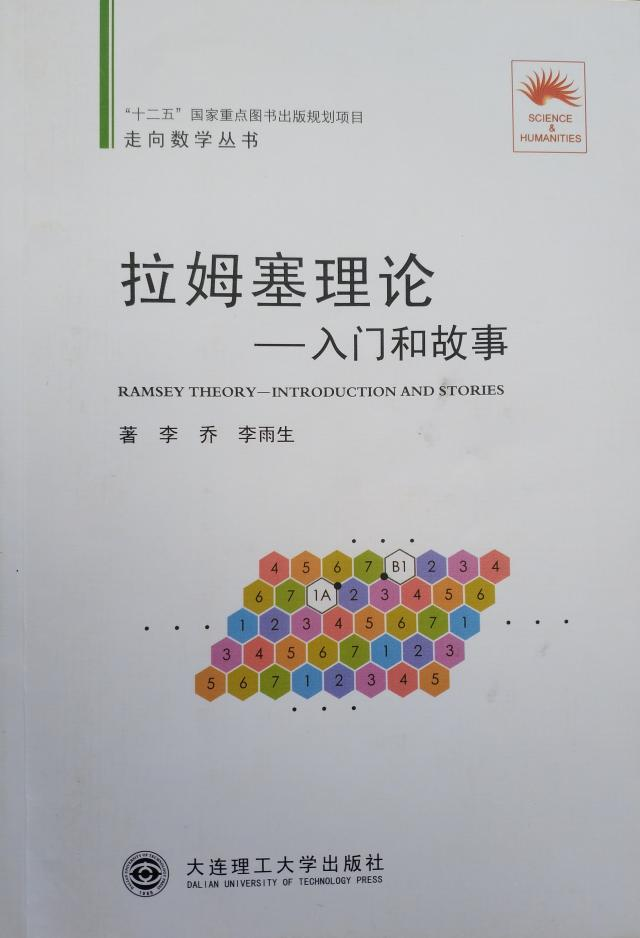• 对于正整数 a, b，定义 [a, b] = {a, a+1, ..., b} 。
• 如果集合 A 中的任何两个元素（可以是相同的）之和都不在 A 中，就说 A 是无和集
• 舒尔数 S(k) 定义为可以分拆为 k 个无和集之并的集合 [1, n] 的 n 的最大可能值。
• S(1) = 1, S(2) = 4, S(3) = 13, S(4) = 44, S(5) = 160, S(6) ≥ 536, S(7) ≥ 1680 。

S(1) = 1 是显然的。

S(2) = 4：集合 [1, 4] 可以分拆为两个无和集：A1 = {1, 4} 和 A2 = {2, 3}，并且只有这么一种分拆方法。又因为 5 = 1 + 4 = 2 + 3，所以 S(2) = 4 。

S(3) = 13：请读者试着不使用计算机证明：S(3) ≥ 13 。

S(4) ＝ 44 是在 1965 年借助计算机求得的。

S(5) = 160 是在 2017 年借助计算机求得的。

参考资料